Function Repository Resource:

# SymbolicFourier

A symbolic version of the Fourier function

Contributed by: Jan Mangaldan
 ResourceFunction["SymbolicFourier"][list] finds the discrete Fourier transform of a list of complex numbers.

## Details and Options

The discrete Fourier transform vs of a list ur of length n is by default defined to be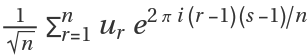.
As with the numeric Fourier function, the zero frequency term appears at position 1 in the resulting list.
Other definitions are used in some scientific and technical fields.
Different choices of definitions can be specified using the option FourierParameters.
With the setting FourierParameters{a,b}, the discrete Fourier transform computed by Fourier is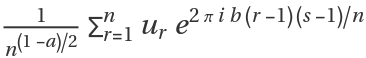.
Some common choices for {a,b} are {0,1} (default), {-1,1} (data analysis) and {1,-1} (signal processing).
The setting b=-1 effectively corresponds to conjugating both input and output lists.
To ensure a unique inverse discrete Fourier transform, |b| must be relatively prime to n.
The list of data supplied to ResourceFunction["SymbolicFourier"] need not have a length equal to a power of two.
The list given in ResourceFunction["SymbolicFourier"][list] can be nested to represent an array of data in any number of dimensions, and does not need to have numeric entries.
The array of data must be rectangular.

## Examples

### Basic Examples (2)

Find a discrete Fourier transform:

 In:=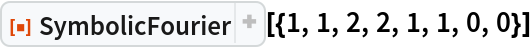Out=Compare with the built-in Fourier:

 In:=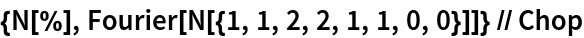Out=### Scope (3)

SymbolicFourier can be used on lists with symbolic elements:

 In:=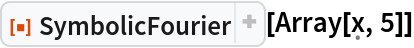Out=Compute the 2D Fourier transform of a symbolic 2×2 matrix:

 In:=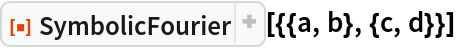Out=Compute the 2D Fourier transform of a section of the Hilbert matrix:

 In:=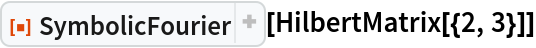Out=x is a rank-3 tensor with nonzero diagonal:

 In:=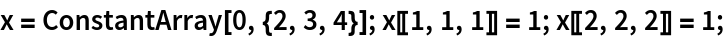Compute the 3D Fourier transform:

 In:=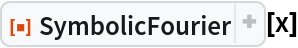Out=### Options (1)

#### FourierParameters (1)

Use FourierParameters to specify a different normalization:

 In:=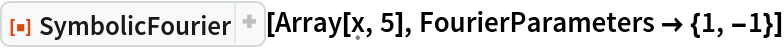Out=### Properties and Relations (2)

Applying SymbolicFourier to a vector is the same as pre-multiplying the vector with FourierMatrix:

 In:=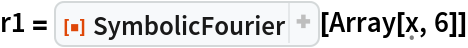Out=In:=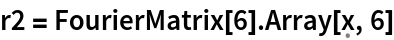Out=In:=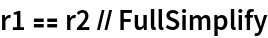Out=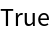The result of SymbolicFourier produces a result in factored form, often with a lower LeafCount:

 In:=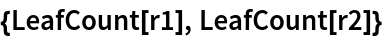Out=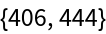## Version History

• 1.1.0 – 06 March 2023
• 1.0.0 – 26 May 2021